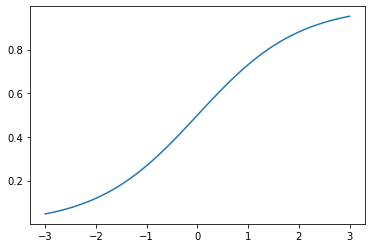# Odds and LogOdds

### Odds

The probabilty of an event occuring is a simple ratio of “instances where it happens” divided by “all possibilities”, or

$\frac{ObservedTrue}{AllObservations}$

For example, rolling a 1 on a 6-sided die is

$\frac{1}{6} = .166667$

By contrast, the odds of an event is a flat look at “instances that it happens” against “instances that it doesn’t happen”. In our dice example, we’d simply have

$1:5$

### Odds Ratio

Alternatively, we could express the odds of an event as a ratio of

$\frac{Pr(Occurring)}{Pr(NotOccurring)}$

This gives us a single number for ease of interpretation– think 199:301 vs 0.661

### Log Odds

However, it should be immediately obvious that interpretation of the scale of the odds ratio leaves something to be desired.

For instance, if something happens with 1:6 odds, the odds ratio is .166. Conversely, if we were looking at 6:1 odds, the odds ratio would be 6.0.

Indeed, if something is more likely to happen, the odds ratio will be some value between 1 and infinity. On the other hand, if it’s less likely, it will simply be bounded between 0 and 1.

This is where the log function proves to be particularly useful, as it gives a symmetric interpretation of two numbers in odds, symmetric around 0.

Taking the log of the above, we’ve got:

1:6 -> 0.166 -> log(0.166) -> -0.77

6:1 -> 6.0 -> log(6.0) -> 0.77

### Log Odds and Logistic Regression

Another useful application of the log odds is in expressing the effect of one unit change in a variable.

Because the logit function has a non-linear shape

%pylab inline

X = np.linspace(-3, 3, 100)
y = 1 / (1 + np.exp(-X))

plt.plot(X, y);
Populating the interactive namespace from numpy and matplotlibone step in the X direction will yield a variable change in y, depending on where you started.

This short video does a great job running through the math of it, but the log odds can be expressed linearly, with resepect to X, as

$\ln(\frac{p}{1-p}) = \beta_0 + \beta_1 X$

Exponentiating both sides, we can see that a unit increse in X is equivalent to multiplying the odds by exp(beta1)

$\frac{Pr(happening)}{Pr(notHappening)} = \frac{p}{1-p} = e^{\beta_0 + \beta_1 X}$